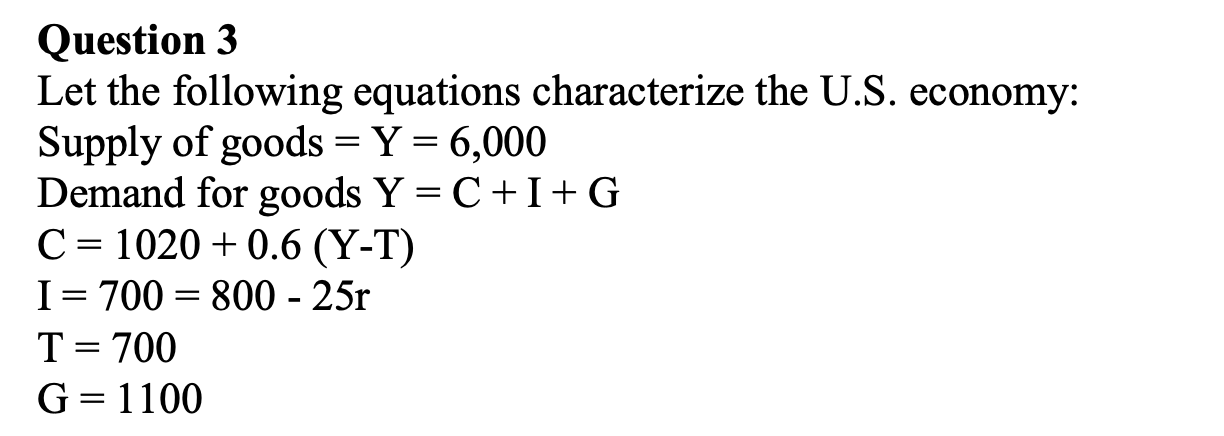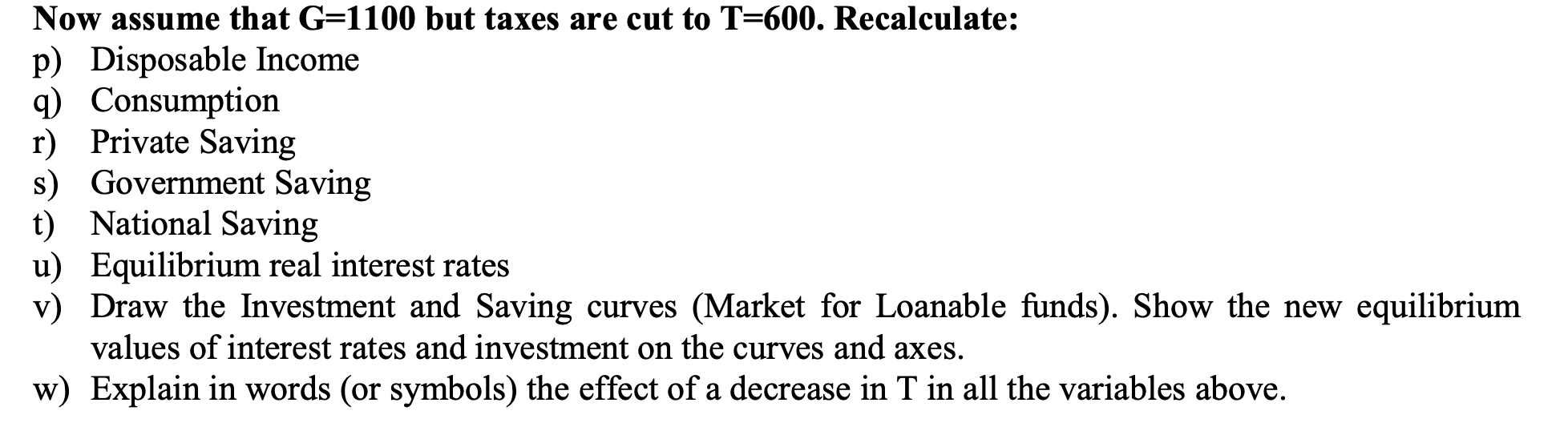# Question 3 Let the following equations characterize the U.S. economy: Supply of goods = Y = 6,000 Demand for goods Y=C+I+G C= 1020 + 0.6 (Y-T) I= 700 = 800 – 25r T= 700 G=1100

Question-AnswerCategory: QuestionsQuestion 3 Let the following equations characterize the U.S. economy: Supply of goods = Y = 6,000 Demand for goods Y=C+I+G C= 1020 + 0.6 (Y-T) I= 700 = 800 – 25r T= 700 G=1100

### Question 3 Let the following equations characterize the U.S. economy: Supply of goods = Y = 6,000 Demand for goods Y=C+I+G C= 1020 + 0.6 (Y-T) I= 700 = 800 – 25r T= 700 G=1100A – Disposable income
= Y – t
= 6000 – 600
= \$ 5400
B – Consumption = 1020 +0.6(5400)
= 1020 + 3240
= \$ 4260
C – Private saving = Y – C
= 6000 – 4260
= \$ 1740
D – Government saving
= T – G
= 600-1100
= \$ (-500)
E – National saving = Private + Government saving
= 1740 -500
= \$ 1240